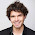## Tuesday, May 28, 2013

### Calculating Diffusion Coefficients from Random Walk Monte Carlo

This is taken from a lecture I gave during the Molecular Statistics course at University of Copenhagen, which I co-teach with +Jan Jensen  and +Jimmy Kromann. The students have to program different types of molecular simulations and are not expected to have any programming experience before the course.

Random walk Monte Carlo seems a bit silly at first glance, since a simulation does not really contain a lot of information. As the name implies, the motion of the particle is completely random, much like Brownian motion of a particle suspended in a fluid. The behavior of a particle in a random walk simulation is very much like the movement of the average particle in a fluid, which randomly and constantly bounces into other particles, every time from a random direction.
A collection on particles will then have average properties much like a liquid or (as we have looked a lot at in the course) the Lennar-Jones fluid. Do note, that  there are no interactions between any particles.

A simple one-particle random walk Monte Carlo simulation is shown here.

The equations of motion are shown here:
$$x(t + dt) = x(t) \pm \mathrm{random.random()} \cdot \mathrm{d}l$$
$$y(t + dt) = y(t) \pm \mathrm{random.random()} \cdot \mathrm{d}l$$

where random.random() is the Python default random number generator and $\mathrm{d}l$ is a scaling factor that determines the average step-length. The fact that the equation does not include any interaction terms makes it computationally cheap to simulate a lot of particles.

In the last Molecular Statistics class we used random walk Monte Carlo to obtain the diffusion coefficient via Fick's second law, here in one dimension:
$$\frac{\partial \phi}{\partial t} = D\ \frac{\partial^2 \phi}{\partial x^2}$$
The solution of Fick's second law is a Gaussian function
$$\phi (x, t) = \frac{1}{\sqrt{2\ \pi\ \sigma^2}} \exp \left( - \frac{x^2}{2 \sigma^2}\right)$$
with $\sigma = \sqrt{2\ D\ t}$. So if we can calculate $\sigma$ from the distribution of particles, we can immediately get the diffusion coefficient.
The simple approach is to start a random walk simulation with many particles and then fit the distribution of particles to a Gaussian.

We used this extremely simple Python/Numpy program to simulate random walk and calculate $D$:

X = numpy.zeros(n_particles)

for i in range(n_steps):
X += numpy.random.uniform(-dl, dl, n_particles)
sigma = X.std()

print "D =", sigma**2 / ( 2 * i)

The simulation looks like this in 2D (with 400 particles):

As you can see, the particles collectively look like they are diffusing through a medium. The diffusion constant is the derived (here only in the $X$-direction by fitting the distribution to a Gaussian function (which was simpy done via Numpy's .std() function). The time evolution of the distribution of particles (blue histogram) and the Gaussian fit (red curve) looks like this:

The time evolution of $D$ should converge pretty quickly. You can see $D$ for a simulation with 10,000 particles below. It took a matter of seconds on Jimmy's old laptop to run this. Imagine doing an long enough MD with 10,000 particles on your laptop!

1.Dear Dr. Christensen,
I read some papers that point out the diffusion coefficient can be calculated by mean square displacement, which is = 2Dt. From you information, the sigma is the standard deviation of distribution of the particles after ran many times simulation. Are the and sigma same?
In addition, if we want to calculate the diffusion coefficient of the system, do we need to set a time for each step? What value of the step time will be appropriate ?
Jian Qiu

2.Sorry, the web cannot recognize the symbol, the mean square displacement is (r2)

3.I have been exploring for a little for any high quality articles or weblog posts in this kind of area . Exploring in Yahoo I ultimately stumbled upon this web site. Reading this info So i am happy to convey that I've a very just right uncanny feeling I discovered just what I needed. I most without a doubt will make sure to do not put out of your mind this site and provides it a glance on a relentless basis.Click on Cloud Hosting LLC to Buy Low cost Hosting

4.This comment has been removed by the author.